Latest Banking jobs   »

# Quantitative Aptitude Quiz For LIC ADO Mains 2023- 05th April

Directions (1-5): The following questions are accompanied by two statements (I) and (II). You have to determine which statements(s) is/are sufficient/necessary to answer the questions.
(a) Statement (I) alone is sufficient to answer the question but statement (II) alone is not sufficient to answer the question.
(b) Statement (II) alone is sufficient to answer the question but statement (I) alone is not sufficient to answer the question.
(c) Both the statements taken together are necessary to answer the question, but neither of the statements alone is sufficient to answer the question.
(d) Either statement (I) or statement (II) by itself is sufficient to answer the question.
(e) Statements (I) and (II) taken together are not sufficient to answer the question.

Q1. An article is marked 35% above its cost price, find the cost price of the article.
Statement I: Discount allowed on article is Rs.190 more than profit earned on the article.
Statement II: Ratio of selling price of the article to marked price of the article is 4 : 5.

Q2. Deepak is 20% less efficient than Dharam. Find efficiency of Shivam.
Statement I: Shivam and Dharam working together can complete a piece of work in 26 2/3 days and Deepak and Shivam working together an complete same piece of work in 28 4/7 days.
Statement II: Deepak is 60% less efficient than Shivam and Dharam working alone can complete a piece of work in 80 days.

Q3. Asif invested Rs.80000 across two schemes – A & B offering SI in the ratio 5 : 3 respectively. Find total interest earned by him.
Statement I: Rate of interest offered by scheme – B is twice of that of offered by scheme – A and period of investment in both schemes is 4 years.
Statement II: Interest received from scheme – B is Rs.6000 more than interest received from scheme – A.

Q4. Average of present age of Aman, Bhanu and Chaman is 34 years. Find present age of Chaman.
Statement I: Aman’s present age is 100% more than Chaman’s present age and ratio of Bhanu’s age 6 years hence to Chaman’s present age is 2 : 1.
Statement II: Sum of Aman’s and Bhanu’s present age is 78 years.

Q5. Ratio of length of train – A and train – B is 4 : 5. Find speed of train – A.
Statement I: Train – A can cross a 500m long platform in 28 seconds and train – A crosses train – B while running in same direction in 54 seconds.
Statement II: Train – B can cross a pole in 15 seconds.

Directions (6-10): The following questions are accompanied by two statements A and B. You have to determine which statements(s) is/are sufficient/necessary to answer the questions.
(a) Statement A alone is sufficient to answer the question but statement B alone is not sufficient to answer the questions.
(b) Statement B alone is sufficient to answer the question but statement A alone is not sufficient to answer the question.
(c) Both the statements taken together are necessary to answer the questions, but neither of the statements alone is sufficient to answer the question.
(d) Either statement A or statement B by itself is sufficient to answer the question.
(e) Statements A and B taken together are not sufficient to answer the question.

Q6. Find the overall profit or loss percentage of retailer if he sells two bats and four balls.
(A) Cost price of 3 bats is equal to selling price of 4 balls while profit percentage on selling one bat is 50%.
(B) On selling two bats retailer earn 50% profit while on selling four balls retailers’ loss 40% of his money.

Q7. A box contains 4 green ball, ‘x’ blue balls, 5 violet balls and ‘y’ yellow balls. Find the value of ‘x + y’.
(A) Probability of choosing two green ball is 1/20.
(B) Probability of choosing one blue ball or one violet ball is ½.

Q8. If w > 1 and n ≠ 0,1 and ‘w’ and ‘n’ both are integers. Is ‘n’ a positive integer?
(A) w^2n>w^n
(B) n^2w > n^w

Q9. Find the number of persons in a group if average weight of the group is 16?
(A) If one person left the group having weight 16 then average weight of the group is same as before.
(B) If two persons join the group having weight 16 and 28, then the average weight of group increases by 2.

Q10. In how many days ‘A’, ‘B’ and ‘C’ together can complete the work?
(A) A is 50% more efficient then B who is 100% more efficient than C while A and B together can complete the work in 12 days.
(B) ‘A’ and ‘B’, ‘B’ and ‘C’ and ‘C’ and ‘A’ together can complete the work in 12, 20 and 15 days respectively.

Directions (11-15): In the following questions, two quantities (I) and (II) are given. You have to solve both the quantities and mark the appropriate answer.

Q11. Quantity I: Anurag invested Rs.3000 more than that of Veer, while ratio of investment period for Anurag and Veer is 5 : 6 respectively. If out of total profit of Rs.27360, Veer got Rs.12960, then find total investment done by Anurag.
Quantity II: Shivam and Deepak started a business by investing Rs.25000 and Rs.10000 respectively. At the end of the year, they decided to divide 60% of the total profit equally and rest in the investment ratio. If they had divided total profit in investment ratio, then Shivam will receive Rs.9000 more profit than that of he received actually, then find the difference between actual profit share of Shivam and that of Deepak?
(a) Quantity I < Quantity II
(b) Quantity I ≤ Quantity II
(c) Quantity I > Quantity II
(d) Quantity I ≥ Quantity II
(e) Quantity I = Quantity II or no relation.

Q12. Quantity I: Aman invested Rs.100000 at 16% p.a. The interest was compounded half yearly in the first year and in the second & third year it was compounded yearly. What will be the total interest received by man at the end of 3 years?
Quantity II: The simple interest received on a sum of Rs.2,30,000 at the rate of 23% p.a. in N years is Rs.4,23,200. Find the CI received on the same sum in (N/4) years at the rate of 11% p.a. compounded annually.
(a) Quantity I < Quantity II
(b) Quantity I ≤ Quantity II
(c) Quantity I > Quantity II
(d) Quantity I ≥ Quantity II
(e) Quantity I = Quantity II or no relation.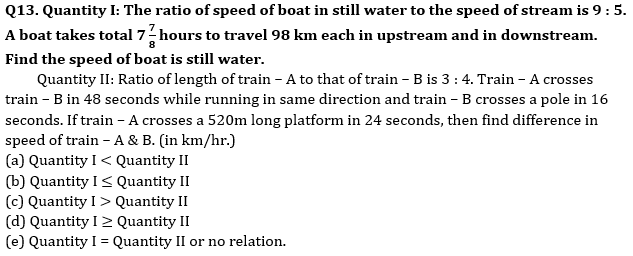Q14. Quantity I: Ratio of cost price of two articles – A & B is 5 : 6 respectively and shopkeeper marked article – A & B 40% and 25% above cost price respectively. If shopkeeper allows discount of 25% on A and 10% on B, then he get a total profit of Rs.300. Find the total cost price of article A & B.
Quantity II: Selling price of an article sold by Amit and Arun is same. Amit sold article at 20% profit and Arun sold at 25% loss. Amit calculated profit percent at cost price whereas Arun calculated loss percent at selling price. If total loss of Amit and Arun together is Rs.200. Find total cost price of Amit’s article and Arun’s article ?
(a) Quantity I < Quantity II
(b) Quantity I ≤ Quantity II
(c) Quantity I > Quantity II
(d) Quantity I ≥ Quantity II
(e) Quantity I = Quantity II or no relation.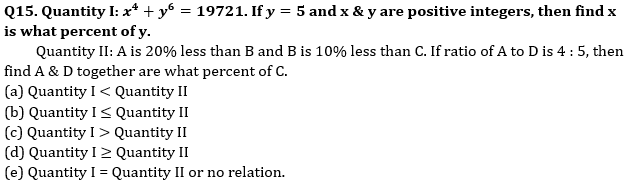Solutions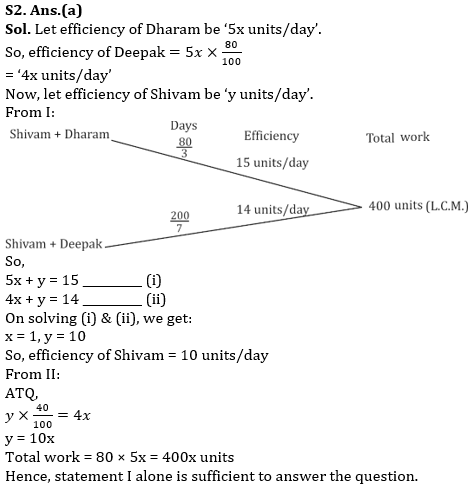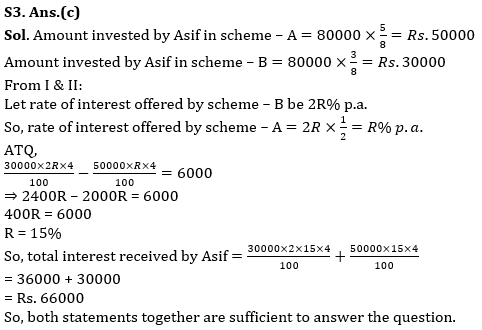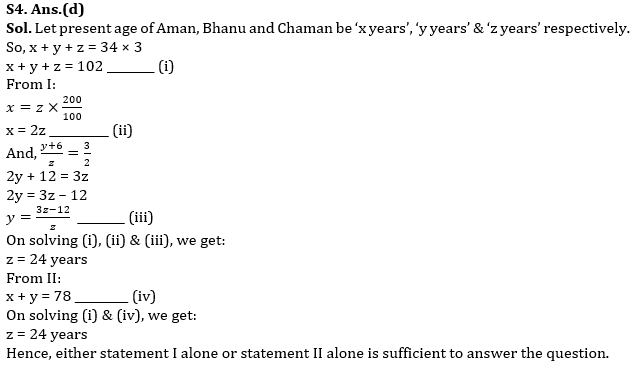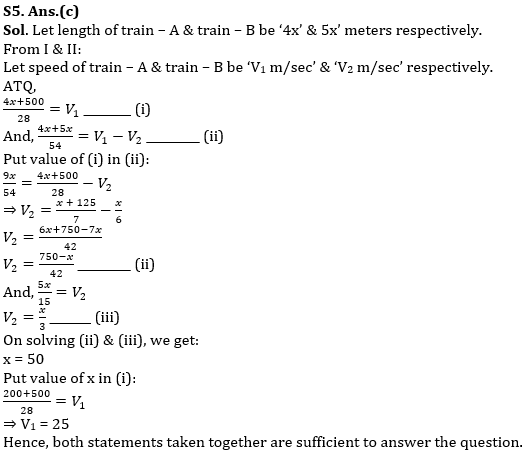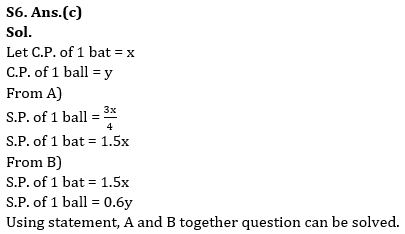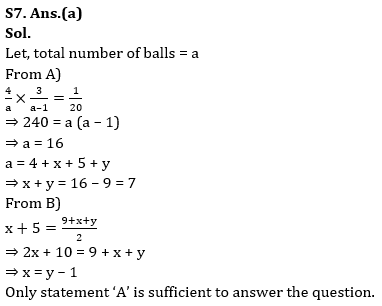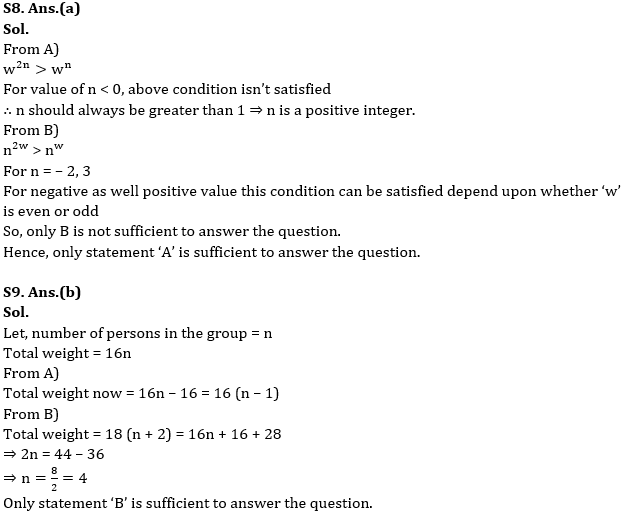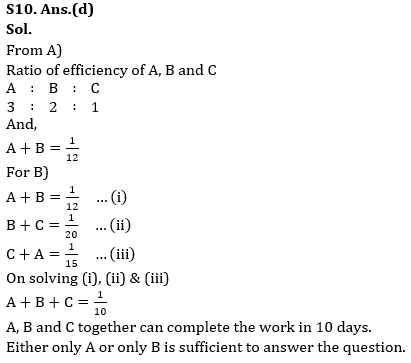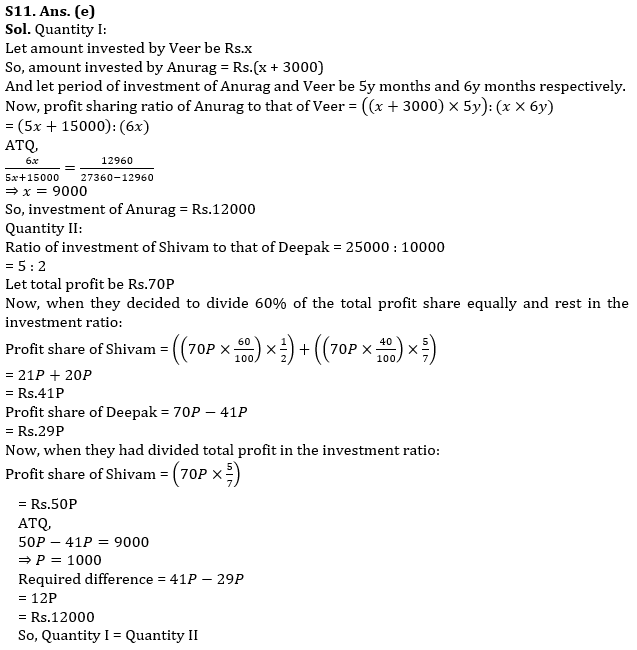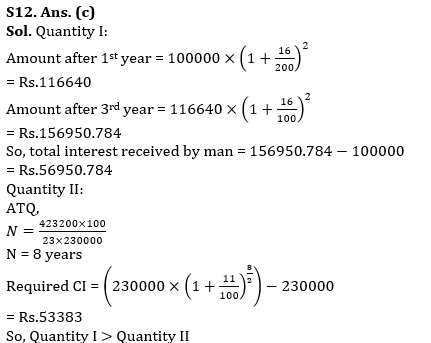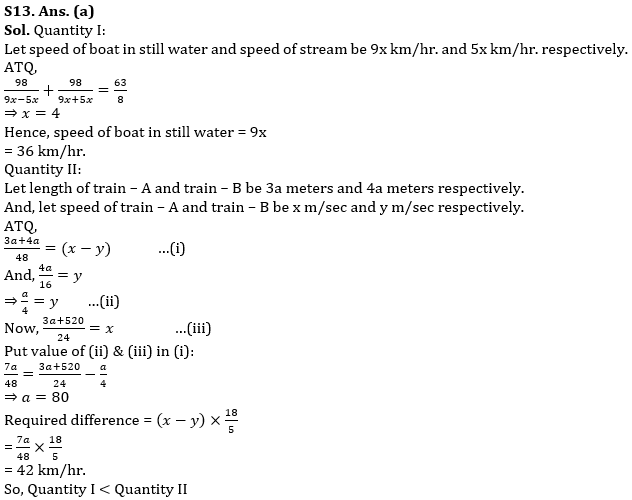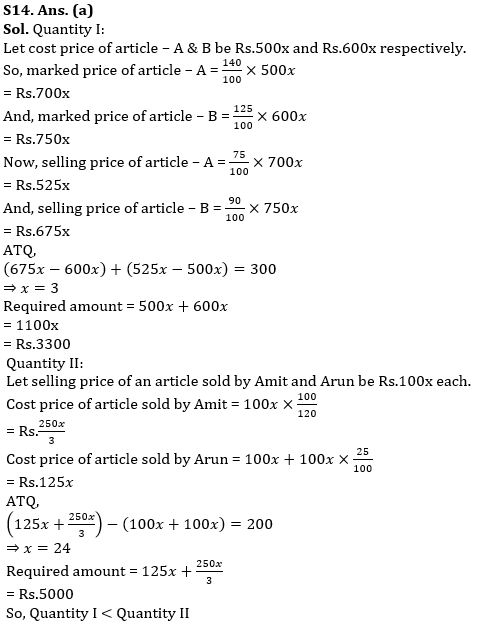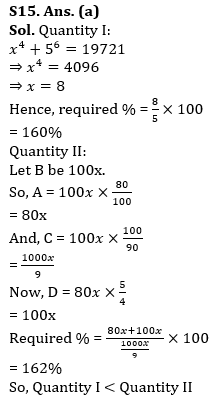## FAQs

### When is the LIC ADO Mains Exam scheduled?

The LIC ADO Mains exam is scheduled to be held on 23 April 2023

#### Congratulations!Union Budget 2023-24: Free PDF